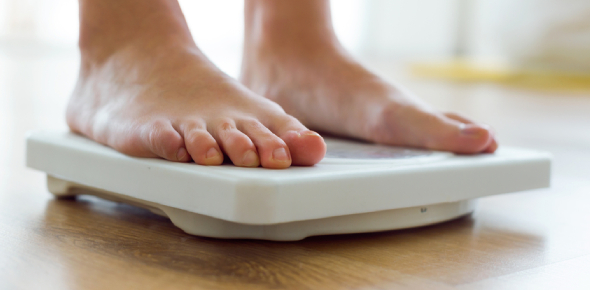# Interesting Weight Quiz: Trivia Questions!

8 Questions | Total Attempts: 90SettingsInteresting weight quiz: trivia questions! Weight is the force exerted to a body by gravity. Do you know that people weigh different when they are on earth when compared to the moon? Do you know that the gravity of the moon is weaker than that on the earth? Do take up the quiz below and see how much you know about the differences and implications to an astronaut. Have fun!

• 1.
An astronaut weighs 700N on earth, what is his weight on the moon?
• A.

43.75

• B.

50.21

• C.

70

• D.

116.66

• 2.
What is his mass on earth?
• A.

100.00

• B.

60.45

• C.

71.42

• D.

85.50

• 3.
What is his mass on the moon?
• A.

71.42

• B.

70.65

• C.

65.10

• D.

53.67

• 4.
A dog weighs 18.75N on the moon what does he weigh on Earth?
• A.

450.76

• B.

375.65

• C.

275.60

• D.

112.50

• 5.
The dogs mass changes from earth to the moon.
• A.

True

• B.

False

• 6.
An astronaut's shuttle weighs 1,223,260.5N on the moon how much on earth.
• A.

1,223,260.5

• B.

354,778.1

• C.

203,876.8

• D.

222,346.7

• 7.
How much does the 100kg astronaut weigh on the moon?
• A.

163 N

• B.

100 N

• C.

53 N

• D.

10 N

• 8.
What is the mass of a 100kg astronaut on Earth?
• A.

980

• B.

600

• C.

100

• D.

50

Related TopicsBack to top